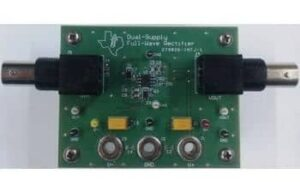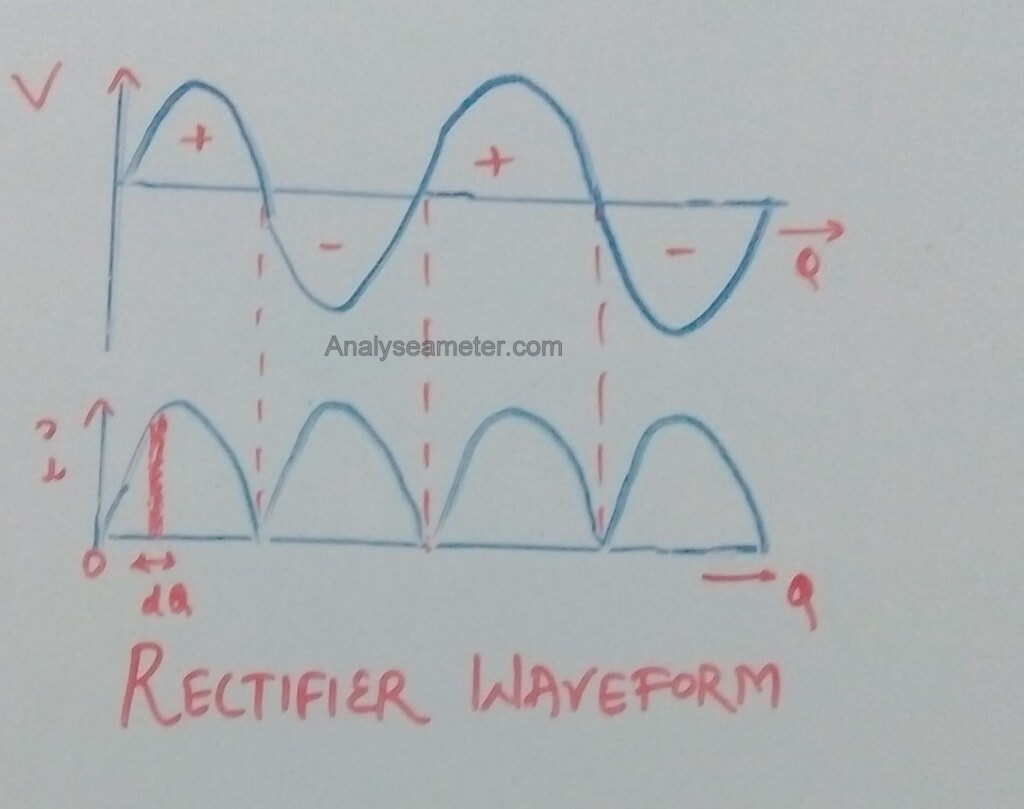# Full wave Rectifier Efficiency Equation and Applications

When I was studying, the question that mostly asked was Efficiency equation of full wave rectifier circuit and where it is used.

Also some other equations like peak factor and form factor was asked with derivation was asked.

And because these equations involves mathematical expressions, this is the part where I and most of the students faced difficulty.

So I decided to include Mathematical derivation of these equations of full wave rectifier also.

We have already covered the characteristics and some basics of Full wave rectifier circuit.## Full-wave Rectifier Equations

Every Rectifier type has its own efficiency and other properties. Basically, there are 3 main equations related to full wave rectifier.

1. Efficiency Equation: Tells us how much AC power or AC voltage is used by this rectifier. The result comes in percentage.
2. Form factor
3. Peak factor

I am going to describe all of these one by one in this article.

### Full-wave Rectifier Efficiency Equation

The efficiency of a full wave rectifier is defined as the ratio of DC power output to the applied input AC power. Therefore, it is given as;

η = DC Power Output / AC power input

Where η is efficiency.

#### Full Wave Rectifier Efficiency Calculation:

Let the AC voltage applied (input voltage to be rectified) is v. And as we have to find maximum efficiency, we will take it as variable and suppose it to be Vm sin θ.

Hence

v= Vm sin θ     [ Vm = Vmax and as the AC voltage is sinusoidal in nature sin θ will very from 0 to 1]

We take rf as the forward resistance of crystal diode and RL be the load resistance of the circuit.

The diagram given below shows the input voltage wave and rectified output wave.The instantaneous value of current is given by the equation:

i = v / (rf + RL)

As we know,

v = Vm sin θ

Therefore,

i = Vm sin θ / (rf + RL)

When sin θ = 1, then current = maximum. Therefore,

Im = Vm / (rf + RL)

Where,

i = Im sin θ

Since output is obtained across RL , therefore

D.C power output = Idc2 RL

= Iav2 RL

Where,

Iav = ʃ (i dθ) / π   ….. (i)

Integrate equation (i) from 0 to π,

Iav = (1 / π) * ʃ Im sin θ dθ

= (Im / π) * ʃ sin θ dθ

= (Im / π) [ – cos θ ]

= (Im / π) [ -(-1-1)]

= 2 (Im / π)

= (2Im / π)

Therefore, DC power output is given as,

Pdc = Idc2 RL = (2Im / π)2 RL

And AC power input is given as,

Pac =Irms2 (rf + RL)

Where,

** Irms =  ʃ (i2 dθ) / π ….. (ii)

= √ (1 / π) * ʃ Im2 sin2 θ dθ

= √ (Im2 / π) * ʃ ( 1- cos 2θ)/ 2  dθ

= √ (Im2 / 2π) * [ ʃ dθ – ʃ cos 2θ dθ ]

= √ (Im2 / 2π) * [[θ] – [sin 2θ / 2]]

= √ (Im2 / 2π) * [π – 0]

= Im / √ 2

Therefore, AC power input is given as,

Pac =Irms2 (rf + RL)

=( Im / √2)2 (rf + RL)

As we know,

Rectifier Efficiency (η) = Pdc / Pac

Put the values of Pdc and Pac from above equations, therefore,

η =[ (2Im / π)2 * RL ] / [( Im / √2)2 * (rf + RL)]

= 0.812 RL / (rf + RL)

= 0.812 / (1+ rf /RL)

If rf is neglected as compared to RL then the efficiency of the rectifier is maximum. Therefore,

η max =0.812 = 81.2%

### Calculation of Form Factor and Peak factor:

#### Form factor of Full wave Rectifier:

It is defined as the ratio of RMS value of the output voltage to the average value of the output voltage. Its equation can be given as:

Form Factor = RMS value of the output voltage / average value of the output voltage

= (Vm / √2) / (2 Vm / π) = π / 2 √2

Form Factor = 1.11

#### Peak Factor of Full wave Rectifier:

It is defined as the ratio of the peak value of the output voltage to the RMS value of the output voltage. Its equation can be given as:

Peak factor = Peak value of the output voltage / RMS value of the output voltage

= Vm / (Vm / √2)

Peak Factor = √2

## Applications of Full wave Rectifier:

Full wave rectifier is of two types, center tap and bridge rectifier. Both these rectifiers are used for following purposes depending upon the requirement.

• It can be used to detect the amplitude of modulated radio signal.
• It can be used to supply a polarised voltage in welding.
• It is mainly used in power supply circuits.
• Audio Amplifiers
• Home Inverters

Q: What is the formula of Full wave rectifier efficiency?

Ans: The formula of efficiency of full wave rectifier is η = DC Power Output / AC power input. Where η is efficiency.

Q: What is the Maximum and minimum efficiency of a Full wave rectifier?

Ans: The maximum efficiency of a full wave rectifier is 81.2%. While minimum efficiency is Zero. Maximum efficiency is obtained when Sin θ is 1 and  Minimum efficiency is when Sin θ is 0.

Q: How do you increase the efficiency of a rectifier?

Ans: The best way to increase the efficiency of rectifier is to use smoothing circuits. Smoothing circuits have smaller ripple value and they also reduce the AC part of the output signal.

Q: What is the form factor of Full wave rectifier?

Ans: The form factor of the full wave rectifier is 1.1. It is the ratio of RMS value of the output voltage to the average value of the output voltage.

Q: What is the Peak factor of Full wave rectifier?

Ans: Peak Factor is the ratio of the peak value of the output voltage to the RMS value of the output voltage. For full wave rectifier it is √2.

Ajay Kumar

Hi readers, Ajay Kumar is graduated in Electronics and Communication Engineering is responsible for running this website. With the knowledge, he has gained, he tries to provide the readers as much of it as he can.

## 13 thoughts on “Full wave Rectifier Efficiency Equation and Applications”

1. choudhary nikhil says:

satisfying…..with this topic
keep growing….

1. Nikhil, I am glad to know that you understand the topic. And Thanks for your Good Wishes. 🙂

2. Raj kumar says:

and keep providing such best explanations.

1. Thank you Raj, for investing your time and commenting. These comments keep us motivated.

3. Sylvester kobby says:

Thanks very much for the lesson

4. Rishitha says:

It’s really a very useful n helpful site……..satisfied with it………

1. Hi Rishitha,
Thanks for stopping by to comment. We are glad that you find our website useful. If you have any question you can ask in a comment.

5. suman says:

thank u so much

1. Ajay Kumar says:

Welcome Suman,
We are glad that you find our website helpful. Share the article with your friends too. This keeps us encourage to produce quality and helpful content. 🙂

Thanks sir

1. Gupta Himanshi says: